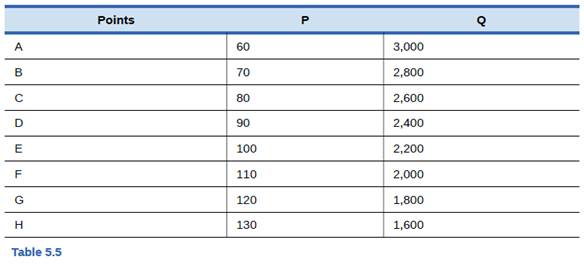Chapter 5, Problem 1SCQ### Principles of Economics 2e

2nd Edition
Steven A. Greenlaw; David Shapiro
ISBN: 9781947172364

#### Solutions

Chapter
Section### Principles of Economics 2e

2nd Edition
Steven A. Greenlaw; David Shapiro
ISBN: 9781947172364
Textbook Problem

# From the data in Table 5.5 about demand for smart phones, calculate the price elasticity of demand from: point B to point C, point D to point E, and point G to point H. Classify the elasticity at each point as elastic, inelastic, or unit elastic.To determine

Calculate the elasticity of demand at various points on the demand curve and classify them as inelastic, unit elastic and elastic.

Price elasticity of demand from point B to C is 0.5

The price elasticity of demand at point D to E is 0.75

The price elasticity of demand at point G to H is 1.32.

Explanation

Let’s calculate the price elasticity of demand from point B to C:

e = ΔQΔP×PQe = 2,6002,8008070×702,800e = 20010×0.025e = (-) -20 ×0.025e = 0.5

Therefore, the price elasticity of demand from point B to C is 0.5. Since the elasticity of demand is less than 1, the demand is inelastic, which means there is less change in quantity demanded as compared to the change in price level.

Now, we find out the price elasticity of demand at point D to E:

e = ΔQΔP×PQe = 2,2002,40010090×902,400e = 20010×0.0375e = (-) -20 ×0.0375e = 0.75

Therefore, the price elasticity of demand from point D to E is 0.75. Since the elasticity of demand is less than 1, the demand is inelastic, which means there is less change in quantity demanded as compared to the change in price level.

Now, we find out the price elasticity of demand at point G to H:

e = ΔQΔP×PQe = 1,6001,800130120×1201,800e = 20010×0.066e = (-) -20 ×0.066e = 1.32

Therefore, the price elasticity of demand from point G to H is 1.32, since the elasticity of demand is greater than 1; the demand is elastic, which means there is large change in quantity demanded as compared to the change in price level.

Concept

Price elasticity of demand: The degree of responsiveness to change in quantity demanded due to change in its price. The formula for calculating elasticity of demand is as follows:

e = ΔQΔP×PQ

#### The Solution to Your Study Problems

Bartleby provides explanations to thousands of textbook problems written by our experts, many with advanced degrees!

Get Started

#### Which one is best?

Foundations of Business (MindTap Course List)

#### REAL RISK-FREE RATE You read in The Wall Street Journal that 30-day T-bills are currently yielding 5.5%. Your b...

Fundamentals of Financial Management, Concise Edition (with Thomson ONE - Business School Edition, 1 term (6 months) Printed Access Card) (MindTap Course List)